# Light: Reflection and Refraction - Class 10

CONTENT LIST

## LIGHT – REFLECTION AND REFRACTION

### Introduction

Light, Which is a form of energy that enables us to see things.

Light originates from a source fall on objects and bounces off . Which are perceived by our eyes and enables us to see the objects.

When light ray travels from one medium to another medium, it undergoes either

• gets absorbed (absorption)
• bounces back (reflection)
• passes through or bends (refraction)

### Reflection of Light

When light rays incident on a polished surface or plane mirror, and return back in the same medium is called Reflection.

Laws of reflection

The law of reflection states that

(i) The incident ray, the reflected ray and the normal ray at the point of incidence, lie in the same plane.
(ii) The angle of incidence is equal to the angle of reflection.

Laws of reflection are applicable to all kind of reflecting surfaces.

### Type of Reflection

The reflection of light can be  categorized into two types :

Specular reflection:
In specular reflection ,  light reflected from a smooth surface at a definite angle.it is also called regular reflection.

Diffuse reflection:
In diffuse reflection ,light reflected  by rough surfaces that tend to reflect light in all directions.It is called irregular reflection.

### Mirror

A mirror is a reflecting surface.When a ray of light is made to fall on the reflecting surface gets reflected according  to law of reflection.

#### Plane mirror

1. Image formed by a plane mirror is always virtual and erect.
2. The size of the image is equal to that of the object.
3. The image formed is as far behind the mirror as the object is in front of it.
4. The image is laterally inverted.

#### Spherical mirror

A spherical mirror is a part of a sphere,  which has curved reflecting surface.

Spherical mirrors have curved surfaces which can be painted on either one of the sides.

There are two types of spherical mirrors :

1. Concave mirror - When an outward surface of a spherical mirror is painted, inward surface acts as reflecting surface then this js called concave mirror.
2.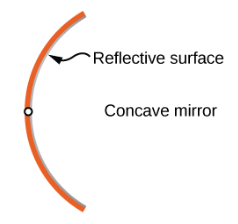3. Convex mirror - When an inward surface of a spherical mirror is painted, outward surface acts as reflecting surface then this is calledConvex mirror.
4.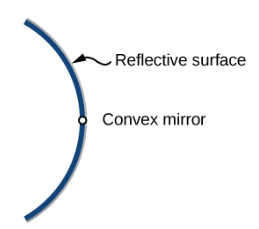### Basic Terms for Spherical Mirrors

There are some common terms that are used while studying spherical mirrors.

#### Pole of mirror

The mid point of the mirror surface is called pole of the mirror. It is represented by the capital letter P. All the measurements are taken from it only so it is treated as an origin for measuring distance.

Centre of curvature:

The reflecting surface of a spherical mirror is a part of a sphere. The center of sphere is called as centre of curvature. It is denoted by the letter C.

The centre of curvature lies outside its reflecting surface and not considered as a part of mirror. The centre of curvature of a concave mirror is in front of mirror. The centre of curvature of a concave mirror is in front of mirror.

The linear distance between the pole and the centre of curvature is known as radius of curvature. Which is represented by the capital letter R, R=2f

Principal axis: An imaginary line that passes through the pole and  centre of curvature of a spherical mirror is called Principal axis . All the measurements are taken on this line.

Aperture: An aperture of a mirror is a point from which the reflection of light takes place or happens. Which gives an idea about the size of the mirror.

Principal Focus: Principal Focus is also called the Focal Point. It is point on the axis of a mirror where the parallel rays of light actualy meet or seems to meet the principal axis after reflection from mirror. Principal Focus of concave mirror is same side of reflecting surface and opposite in convex mirror.

### Image Formation by Spherical Mirrors

Ray Diagrams for a Concave Mirror

1. Object is positioned at infinity:Images formed are highly diminished, point sized and real and inverted at Focus.
2.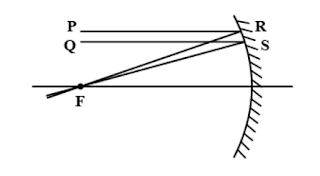3. Object is positioned between infinity and center of curvature(c): Image formed between principal focus (f) and center of curvature (c), diminished , real and inverted.
4.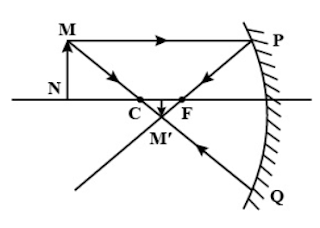5. Object is positioned at Center of Curvature (c): Image formed at the center of curvature, the image is the same size as the object and images are real and inverted
6.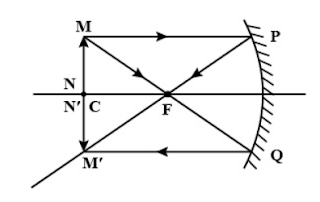7. Object is positioned between the center of curvature (c) and principal focus (f) : Image is formed beyond the center of curvature (c), and the image is real and inverted
8.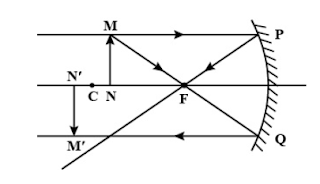9. Object is positioned at principal focus (f):Images formed are highly enlarged images ,real and inverted .
10.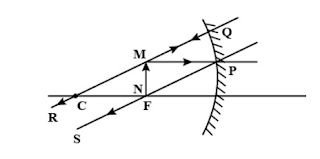11. Object is positioned between principal focus (f) and pole (p):Theimages formed here are formed behind the mirror, images are highly enlarged, images are virtual and erect
12.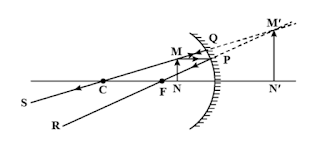Ray Diagrams for a Convex Mirror

1. Object is positioned at Infinity : The images formed are formed at the principal focus (f) behind the mirror and are highly diminished, the images are virtual and erect.
2.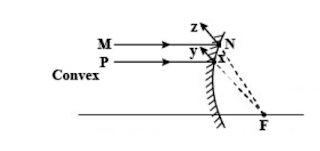3. Object is positioned between the pole (p) and the principal focus (f): The images formed here are formed behind the mirror, between the pole and principal focus (f), the images are diminished and are virtual and erect.
4.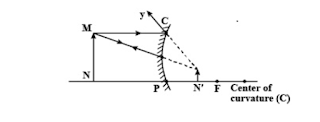#### Uses of convex mirrors

• Convex mirror is used in vehicles as rear view mirrors.
• Convex mirror are used for security purposes, such as in ATMs.
• They are used in sunglasses.
• They are also used as street light reflectors.

Note: The convex mirror always creates a virtual image of the object and the image formed by the convex mirror is smaller than the size of the actual object.

#### Uses of concave mirrors

• Concave mirroris used as a shaving mirror.
• Concave mirror  is used as reflector in torch, head light of automobiles etc.

### Sign Convention for Reflection by Spherical Mirrors

All distances are measured from the pole  of the mirror. Distances measured along the direction of the incident ray are taken  positive. Distance measured along opposite the direction of the incident ray are negative. All distances measured above the principal axis are taken as positive. All distances measured below the principal axis are considered as negative.

### Mirror Formula and Magnification

1/f = 1/v + 1/u

This formula represents the relationship between the distance of the object (u), the distance of the image (v), and the focal length (f) of a Spherical Mirror.

The size of the image formed by any mirror is called Magnification. Image formed by mirror will be magnified, diminished, or equal to the object.

Magnification represents the ratio of the height of the image to the height of the object. which is denoted by “m”.

Therefore, m=h′/h

h′ = the height of the image, h = the height of the object.

Magnification is equal to the negative of the ratio of the image distance(v) to object distance(u).

m = −v/u

### Refraction of light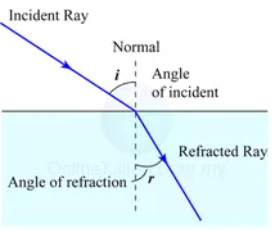Refraction is the bending of light  when light ray passes from one transparent substance into another. This change of direction of light is caused by a change in speed.

The amount of bending depends on two factors:

• Change in speed – change in speed of light rays occur at the surface of interface of two medium. When light rays move from rarer medium to denser ,light rays bend toward the normal and when light rays move from denser to rarer medium ,it bend away from normal.
• Angle of the incident ray – if the light ray enters the substance at a greater angle, the amount of refraction will also be more.
• If the light is entering the new substance from straight on (at 90° to the surface), the light will still slow down, but it won’t change direction at all.

The following are the laws of refraction of light.

1. The incident ray, the refracted ray and the normal to the interface of two transparent media at the point of incidence, all lie in the same plane.
2. The ratio of sine of angle of incidence to the sine of angle of refraction is a constant, for the light of a given colour and for the given pair of media. This law is also known as Snell’s law of refraction. (This is true for angle 0 < i < 90°) If i is the angle of incidence and r is the angle of refraction, then, sini / sinr = constant. This constant value is called the refractive index of the second medium with respect to the first.

#### Refraction through a Rectangular Glass Slab

Change in speed of light rays occur at the surface of interface of air and glass. When light rays move from air medium to glass, light rays bend toward the normal and when light rays move from glass to air, it bend away from normal.

#### The Refractive Index

Refractive Index is a measure of the reduction in the velocity of light in a medium.

Consider a ray of light travelling from medium 1 into medium 2.
Let v1 be the speed of light in medium 1 and v2 be the speed of light in medium 2.

The refractive index of medium 2 with respect to medium 1 is given by the ratio of the speed of light in medium 1 and the speed of light in medium 2.

This is usually represented by the symbol n21. This can be expressed in an equation form as
n21 = Speed of light in medium 1 / Speed of light in medium 2 = v1/v2

If medium 1 is vacuum or air, then the refractive index of medium 2 is considered with respect to vacuum. This is called the absolute refractive index.

### Spherical Lense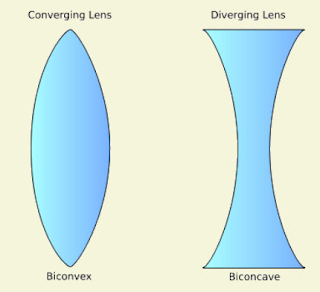Lense is a piece of glass or other transparent material with curved sides used for converging or diverging light rays due to refraction. Spherical lenses are formed by connecting two spherical transparent surfaces.

There are two kind of spherical lenses.

1. Convex lense
2. Lenses formed by binding two spherical surfaces bulging outward are known as convex lenses.It is also called as converging lense.

3. Concave lense
4. Lenses formed by binding two spherical surfaces with inward curved  are known as concave lenses. It is also called diverging lense.

#### Terms Related to spherical  Lense

Pole: It is the centre of the spherical refracting surface of the lens. At this point , the principal axis meets the surface of the lens.

Centre of Curvature: A lens is made up of two spherical surfaces, these two spherical surfaces are a part of a sphere. The centre of these spheres is called as the centre of curvature. Lenses have two Centre of Curvature.

Principal axis: It is an imaginary line passing through the centres of curvature and the pole.

Aperture: Aperture is the area of  lens suitable for refraction is called Aperture.

Focus: It is the point on principle  axis of lense, where light rays  parallel to the axis meet or appear to meet  after refraction.

Focal length: The focal length refers the distance between the optical centre and the focal point or focus of the lens.

#### Magnification

When an object is placed perpendicularly to the principal axis of lens, a image is formed perpendicular to the principal axis due to the refraction of the lense. The size  portion and nature of the image formed depend on the position of the object with respect to the lense.

The ratio of the height of the image to the height of the object is called the magnification of the lens.

m = height of the image/ height of the object

#### Power of a Lens

The power of the lens is taken as the reciprocal of its focal length.

The S.I unit of power is Dioptre.